Thermodynamics and Propulsion

# 15.3 Enthalpy of formation

The systems we have worked with until now have been of fixed chemical composition. Because of this, we could use thermodynamic properties relative to an arbitrary base, since all comparisons could be made with respect to the chosen base. For example, the specific energyfor steam. If there are no changes in composition, and only changes in properties of given substances, this is adequate. If there are changes in composition, however, we need to have a reference state so there is consistency for different substances.

The convention used is that the reference state is a temperature of(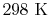) and a pressure of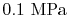. (These are roughly room conditions.) At these reference conditions, the enthalpy of the elements (oxygen, hydrogen, nitrogen, carbon, etc.) is taken as zero.

The results of a combustion process can be diagrammed as in Figure 15.1. The reactants enter at standard conditions; the combustion (reaction) takes place in the volume indicated. Downstream of the reaction zone there is an appropriate amount of heat transfer with the surroundings so that the products leave at the standard conditions. For the reaction of carbon and oxygen to produce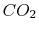, the heat that has to be extracted is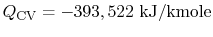; this is heat that comes out of the control volume.There is no shaft work done in the control volume and the first law for the control volume (SFEE) reduces to: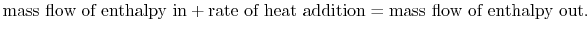We can write this statement in the form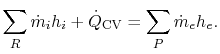(15..1)

In Eq. (15.1) the subscripts R'' and P'' on the summations refer to the reactants () and products (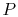) respectively. The subscripts on the mass flow rates and enthalpies refer to all of the components at inlet and at exit.

The relation in terms of mass flows can be written in molar form, which is often more convenient for reacting flow problems, by using the molecular weight,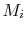, to define the molar mass flow rate,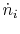, and molar enthalpy,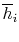, for any individual ith (or eth) component as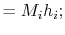The SFEE is, in these terms,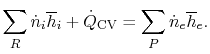(15..2)

The statements that have been made do not necessarily need to be viewed in the context of flow processes. Suppose we have one unit of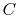and one unit of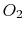at the initial conditions and we carry out a constant pressure reaction at ambient pressure,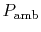. If so,since. Combining terms,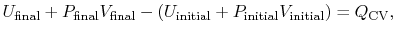or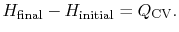In terms of the numbers of moles and the specific enthalpy this is(15..3)

The enthalpy of, atand, with reference to a base where the enthalpy of the elements is zero, is called the enthalpy of formation and denoted by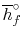. Values of the heat of formation for a number of substances are given in Table A.9 in SB&VW.

The enthalpies of the reactants and products for the formation ofare: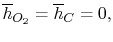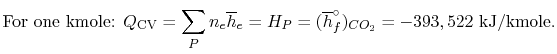The enthalpy ofin any other state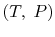is given byThese descriptions can be applied to any compound. For elements or compounds that exist in more than one state at the reference conditions (for example, carbon exists as diamond and as graphite), we also need to specify the state.

Note that there is a minus sign for the heat of formation. The heat transfer is out of the control volume and is thus negative by our convention. This means that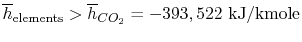.

Muddy Points

Is the enthalpy of formation equal to the heat transfer out of the combustion during the formation reaction? (MP 15.4)

Are the enthalpies ofand(monoatomic hydrogen) both zero at? (MP 15.5)

UnifiedTP